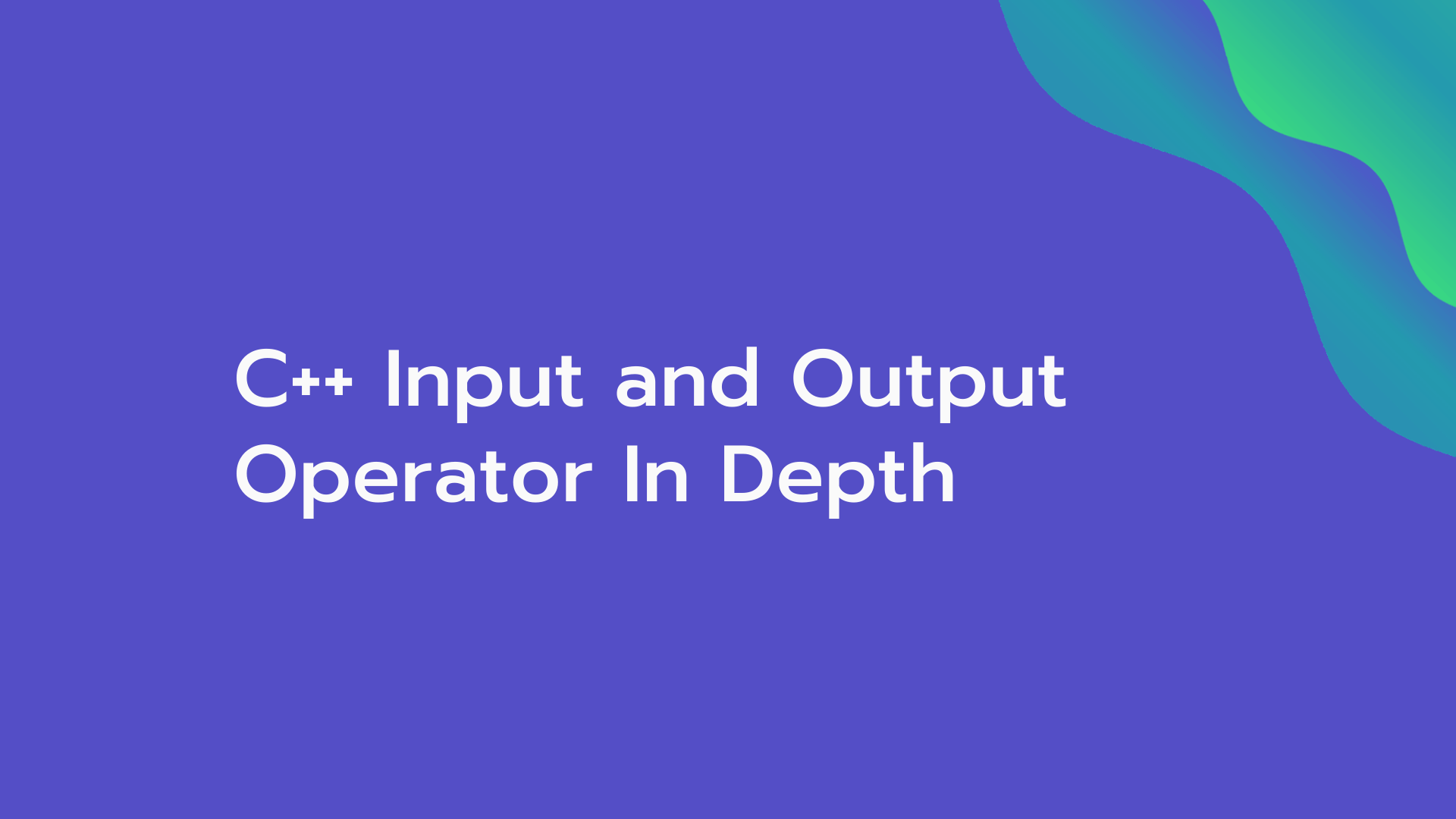# C++ Input and Output Operator In Depth

## Input in C++ is obtained by the user's terminal using a predefined header file iostream and using its object named cin. Input obtained from the user's terminal is known as standard input. The output is displayed using a predefined header file and its object cout.In this tutorial, we will be covering everything you need to know about Input and Output operator in C++. It is very important to learn about taking user input and to display it. It is something that every programmer must learn while learning any programming language.

Input and output operations are done using istream (input stream) and ostream (output stream) classes. If you are what does stream means in C++ so here is the quick information about the stream. In C++ all the devices are treated as files which means all the standard input device (the keyboard) and standard output device are treated as a file and these files are interpreted simply as a sequence or a stream of bytes.

## Output Operator  "<<" :

The output operator in C++ is  ("<<") which means "put to", also called a stream insertion operator. It is used to direct value to standard output. We display output using the combination of cout and "<<". Stream insertion operator (">>") signifies that insert the value that follows into the stream named cout.

### Example:

``````cout<<"My name is Digital Deal Solution"<<endl;
cout<<"My age is"<< 10+9;
cout<<"\nI love food";``````

### Output-

My name is Digital Deal Solution

My age is 19

I love food

Note: We use  "endl" and "\n" to insert a new line in our output.

## Input Operator ">>":

The input operator in C++ is  (">>") which means "get from", also called a stream extraction operator. It is used to read the value from the standard input. We take input using the combination of cin and ">>". Stream extraction operator (">>") signifies "extract the next value" from the stream named cin and assign it to the next named variable.

### Example:

``````int a;
cin>>a;``````

The above-given example will take input of integer type and store it in a variable 'a'. Variable refers to a storage area whose value can be changed during processing.

Important Note: We need to use std is a namespace. Namespaces were added to C++ STD 90. In older versions of C++, since there was no namespace, In older versions of C++ used cout and but in modern C++ we will use std::cout for output and std::cin for input. We just need to add "std::" in front of "cin" or "cout". We will learn more about using namespace in future tutorials.

Let us understand more about taking input and displaying output with the help of a simple example. We will write a program to print the multiplication of two values.

``````//Program to print multiplication of two values
int main() //main function
{
int a,b; // varible declaration of type integer
std::cout<<"Enter the value of a and b respectively"<<std::endl; //display string
std::cin>>a>>b; //taking input
std::cout<<"Product of a and b is "<<a*b; //display string and result
return 0;
}
``````

5 and 6

### Output :

Enter the value of a and b respectively
5
6
Product of a and b is 30

In the above program, we used multiple input and output operators in one statement which is called cascading of I/O operators.

Now here quick tip if you are cosidering writing "std::" every time you use cout, cin, and endl, etc. Then we have a solution for it. We need to add the statement " using namespace std" is our code then we don't need to use std every time we use cout, cin, and endl, etc but we highly recommend you using "std::"  for large programs or projects. Let's have look at the same example using namespace.

``````//Program to print multiplication of two values
using namespace std;
int main() //main function
{
int a,b; // varible declaration of type integer
cout<<"Enter the value of a and b respectively"<<endl; //display string
cin>>a>>b; //taking input
cout<<"Product of a and b is "<<a*b; //display string and result
return 0;
}
``````

The above program will work same as Program 1.

We will stop here and learn more concepts in the next tutorials. We wish you best of luck for future tutorials and if you have any doubt you can ask in the comment box below.0000000